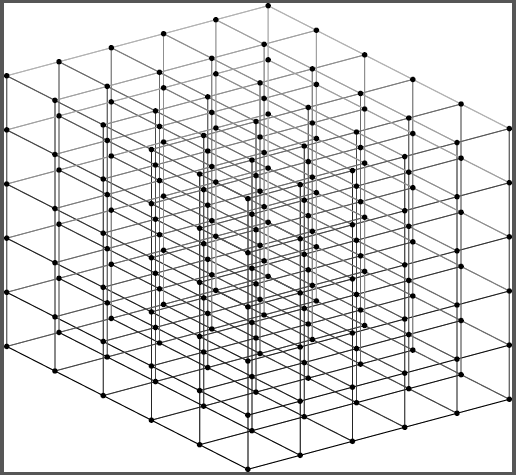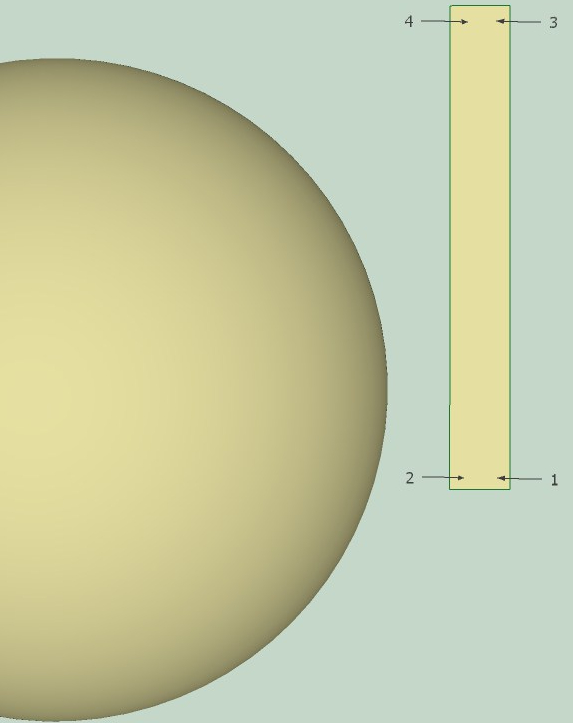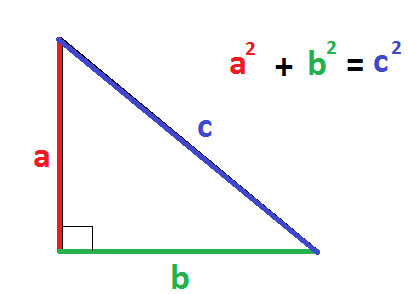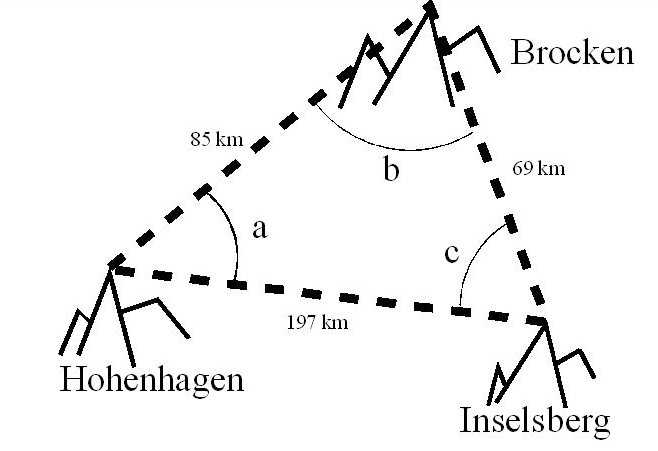## On the curvature of beer coasters and spheres

I told a friend of mine, Josh, about the course I am teaching at Amsterdam University College. I told Josh that in last week’s class we treated Albert Einstein and his relativity theories, and how whoppingly strange it is to imagine curved space (or even spacetime).  At some point during that lecture, as I was calmly and comfortably rambling on about how Einstein’s discoveries had flabbergasted his contemporaries and radically changed our views on reality, one of the students raised her hand: “What are we talking about here? I know what a curve is, that’s when the road bends before me, like a line that’s not straight. But that line is on paper… what does it even mean to say that ‘space itself is curved’?

When the girl had asked her question an indistinct murmur rose up from the group of students. I was silent for a moment, and then I suddenly realised how silly I had been: Here I was, trying to explain how amazing were Einstein’s theories about spacetime and the way it curves, and I had forgotten to start with explaining what this curvature is!

One thing at a time. Let’s first discuss space and spatial curvature. Let’s begin by trying to answer this question: How can it be that space is curved if it has nothing to curve into? how can space bend if it can’t bend anywhere?!

### What is space? Let’s start 3DFig 1. 3D grid with points as a representation of 3D space

We can think of familiar 3D space as a regular three-dimensional grid with points on it (see figure 1). Each of these points represents a location in space where an object can be. We call this space three-dimensional because any point in it has three coordinates.

### ‘Curvature’ is a Misnomer

When I talked about the curvature of space during my lecture, another one of the students asked “if space were curved, shouldn’t we see it curve up or down (like a hill), or to the side (like a corridor in a building)?”

“Well,” I told her, “‘Curvature’ is somewhat of a misnomer I’m afraid.” “In the cases that you mention there are (visible) surfaces that curve (the side of the hill/the walls of the corridor), into a higher dimension. For example, we speak of a hill when the surface of the earth rises above an otherwise horizontal plane in a direction that is perpendicular to the horizontal plane itself.”

“The curvature that Einstein was talking about is something different. Einstein’s theory holds that space itself is curved. But outside space there is nothing (at least, that’s what Einstein believed), so there is nowhere to curve into! how do we make sense of that?”Fig 2. In relativity theory, space is curved around massive objects (like earth). It’s as if the grid that represents space becomes denser in those areas.

“The secret here is to distinguish between extrinsic and intrinsic curvature. Extrinsic curvature requires an extra spatial dimension for its description (like the surface of the hill that we just discussed), whereas intrinsic curvature doesn’t. In the context of the 3D grid in figure 1 we can imagine intrinsic curvature as an increase of the density of gridpoints in a certain area” (see figure 2).

“Now you see why ‘curvature’ is a bit of a misnomer. It isn’t really wrong, but it is misleading. Perhaps it would be better to talk about the stretching and denting of space.”

### Intrinsic Curvature

All this time Josh had been listening to my story. Sipping from his beer, smiling about my educational blunder, and occasionally frowning because of an all-too-hasty step in one of my arguments. Being himself an astronomer, nothing of what I said was really new to him.Fig 3. Dutch pride: a Heineken beer coaster.

“What I’d like to know,” said Josh, “is how you would explain the idea behind intrinsic curvature in an intuitive manner.” “I mean, I understand the math behind it all, but do you think students can get an idea of what intrinsic curvature is without knowing the math behind it? You’re not teaching them any math, are you?” “Then how do you explain the difference between extrinsic and intrinsic curvature?”Fig 4. A line on the surface of a sphere, A, is stretched out into line B. Are A and B equally long?

I was silent for a while, and then I picked up the beercoaster that lay before me. “Suppose we draw a line along the circumference of this beercoaster,” I said while running the top of my finger along a quarter of the coaster’s outline, “If we stretch out this line it will have a length of$frac{1}{4}$ the circumference of the coaster” (figure 3).

“Now compare this to any situation that we actually encounter in physics.” “All objects in the universe are three-dimensional, not two dimensional.”

“Ok,” said Josh, “of course, matter in the universe consists of particles, not of points.” “but what does that matter for our line on the beercoaster? Lines A and B are equally long, whether they represent the path of a point or that of a particle.”

“That’s because the circumference of a circle (or the surface of a cylinder) is only extrinsically curved, and not intrinsically.” “Suppose that we look at a line along the surface of a sphere. In case of a line, the story is essentially the same as in the case of the beercoaster. Any path from the equator to one of the poles of the sphere would do the trick. If we would straighten it, it would have a length of$frac{1}{4}$ the circumference of the sphere.” “The story changes if the path denoted by A and B is that of an object with non-zero width, such as a particle. In that case the path will become a stripe instead of an infinitely thin line. And what will happen to the stripe when the particle moves up the sphere, following its curvature?”Fig 5. When you project a stripe on a sphere the shape of the stripei changes if it is to follow the curvature of the sphere exactly.

“If the stripe represents the path of a particle with non-zero width as it moves from the equator to one of the poles along the sphere, then the bottom corners of the stripe (1 and 2 in figure 5) are points on the equator of the sphere which represent the sides of our particle. They’ll have a distance in between them that is equal to the width of the stripe. If the stripe, when it is wrapped around the sphere, is to follow the sphere’s surface exactly, its two upper corners (3 and 4 in figure 5) have to coincide at the sphere’s north pole!

That’s what intrinsic curvature is all about. The surface of the sphere is intrinsically curved, so the distance between points changes if we move from place to place on the sphere.

### Pythagoras

A nice way to illustrate this is by looking at the shapes of triangles. Consider two things that we know about triangles on a flat surface (mathematicians call such a surface ‘Euclidean’):

1. For the sides of a triangle in which one of the angles is 90 degrees Pythagoras’ theorem holds:$a^2+b^2=c^2$.
2. The inner angles add up to 180 degrees.Fig 6. Pythagoras’ theorem.

On the surface of a cylinder (which we think of as curved because it bends into a dimension not on the surface) Pythagoras’ theorem holds, and the inner angles add up to 180 degrees, no matter how large or small the cylinder. On the surface of a sphere, on the other hand, Pythagoras’ theorem no longer holds, and the inner angles will add up to more than 180 degrees (see figure 7).Fig 7. A triangle on a sphere (left) and in flat space (right).

But wait a minute! Didn’t we say earlier that the difference between extrinsic and intrinsic curvature lies in the additional spatial dimension – a dimension which a surface can curve into? Both in the case of the surface of a cylinder and the surface of a sphere we talk about a dimension that is not on that surface (the radius of the cylinder/sphere), right? So why aren’t they the same kind of curvature?

You’re right. We do use an additional spatial dimension both in the case of the cylinder and that of the sphere. The point, however, is not that we haven’t used an extra spatial dimension to show that a sphere’s surface is curved; but rather that we didn’t need the extra spatial dimension to be able to describe the curvature of the cylinder. We might have just said that Pythagoras’ theorem should be changed in a particular way, or that the inner angles of triangles add up to a number other than 180.

Now we go back to the 3D grid-space that we live in. If Einstein is right, and space is really intrisically curved (as in figure 2), then we should be able to measure that (we are talking science, after all). So how come we don’t see this? For example, if we draw a triangle in the sand on the beach, how come Pythagoras’ theorem always seems to hold?

You guessed it: the curvature of space that is predicted by Einstein can only be detected on a very large scale. Triangles on the beach are simply too small.Fig 8. Gauss’ triangle measurement (1820s)

It might come as a surprise to you, but Einstein wasn’t the first to talk about the curvature of space. The early 19th century physicist and mathematician (a.k.a. all-round smarty pants) Carl Friedrich Gauss tried to measure the curvature of space on a scale much larger than your average beach: he studied a triangle between three mountain-tops and tried to determine whether the inner angles add up to 180 degrees. Regrettably for Gauss, Einstein’s curvature of space manifests itself only on very large scales. Gauss’ triangle was too small, and so he measured angles adding up to 180 degrees. Kudos to Gauss for trying such an amazing experiment, though.  Only by looking deep into the universe with the help of modern technology have we confirmed Einstein’s predictions: space is indeed curved.1.Lindert Benedictus says: